### IMO Shortlist 2009 problem C7

Kvaliteta:
Avg: 0,0
Težina:
Avg: 9,0
Let$a_1, a_2, \ldots , a_n$ be distinct positive integers and let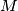$M$ be a set of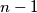$n - 1$ positive integers not containing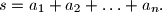$s = a_1 + a_2 + \ldots + a_n.$ A grasshopper is to jump along the real axis, starting at the point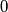$0$ and making$n$ jumps to the right with lengths$a_1, a_2, \ldots , a_n$ in some order. Prove that the order can be chosen in such a way that the grasshopper never lands on any point in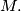$M.$

Proposed by Dmitry Khramtsov, Russia
Izvor: Međunarodna matematička olimpijada, shortlist 2009